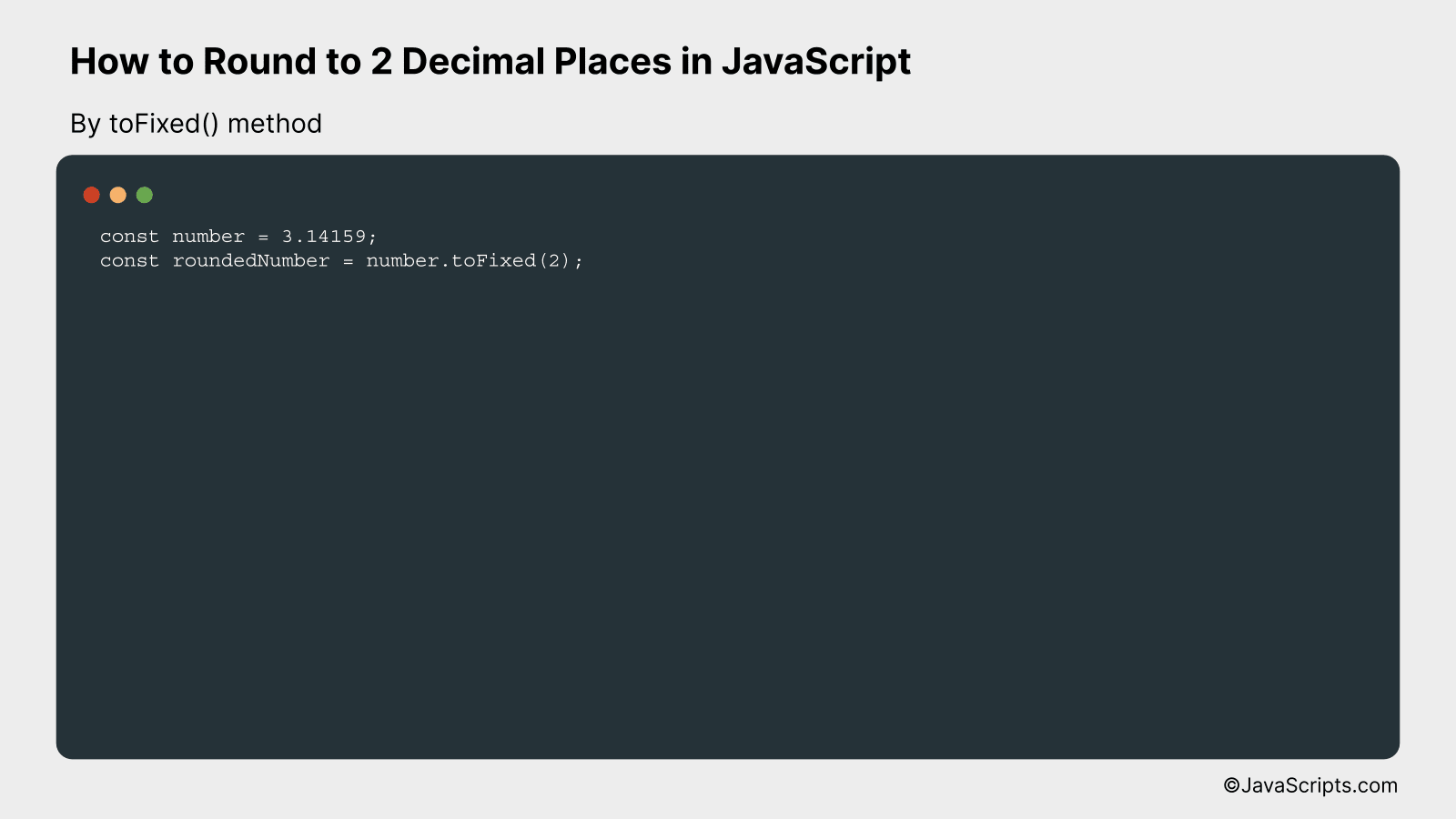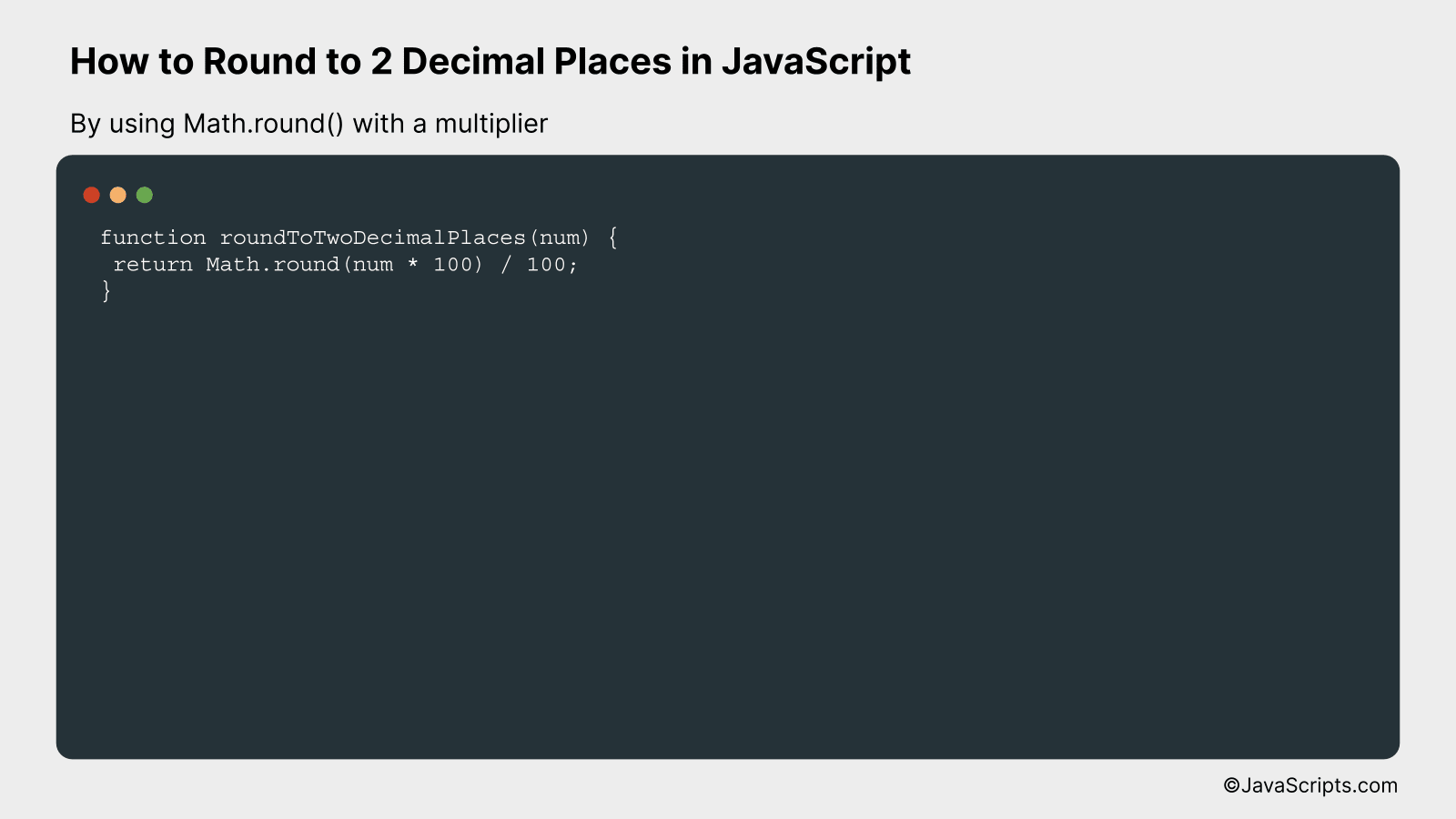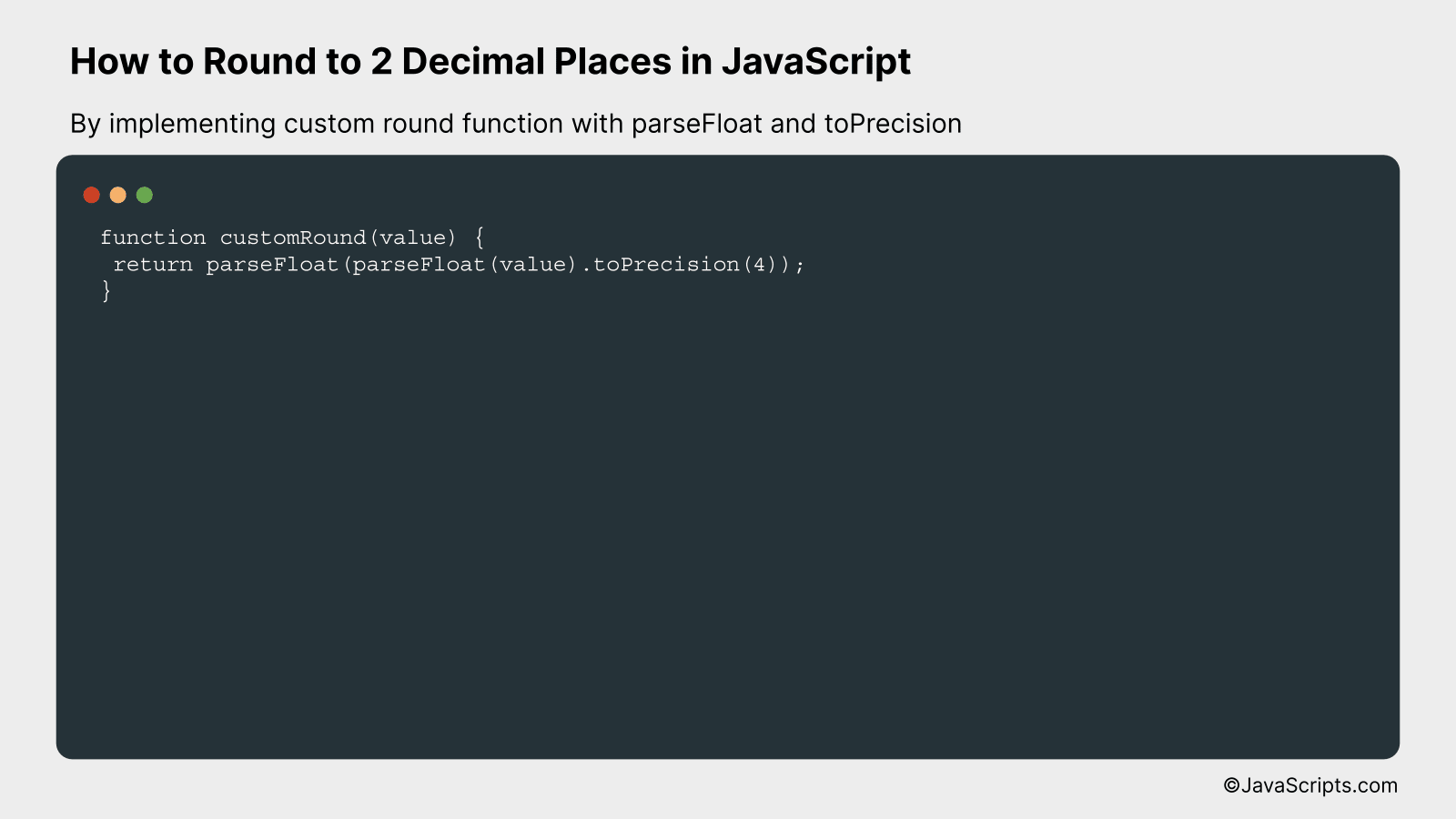# How to Round to 2 Decimal Places in JavaScript

Dealing with decimals in JavaScript can often seem like a daunting task, especially when you have to round them to a specific place. Fear not, my friend. Learning how to precisely round numbers to two decimal places can make your coding journey significantly easier and your output considerably more professional.

Are you a novice programmer or an expert seeking to refresh your skills? Either way, this guide is for you. Together, we’ll tackle the mystery of rounding in JavaScript and break it down into simple, manageable steps. Let’s dive into the pool of decimal numbers!

In this post, we will be looking at the following 3 ways to round to 2 decimal places in JavaScript:

• By toFixed() method
• By using Math.round() with a multiplier
• By implementing custom round function with parseFloat and toPrecision

Let’s explore each…

## #1 – By toFixed() methodTo round a number to two decimal places in JavaScript, you can use the `toFixed()` method, which formats a number as a fixed-point notation string and returns the result. For example, rounding 3.14159 to 2 decimal places will result in 3.14.

``````
const number = 3.14159;
const roundedNumber = number.toFixed(2);
``````

### How it works

The `toFixed()` method operates on a number and rounds it to a specified number of decimal places, converting it to a string in the process.

• Declare a variable `number` and assign the value 3.14159 to it.
• Call the `toFixed(2)` method on the `number` variable, specifying 2 as the desired number of decimal places.
• Store the returned string, which contains the rounded number, in a new variable called `roundedNumber`.
• The resulting string in the `roundedNumber` variable will be “3.14”. You can convert it back to a number using `parseFloat()` or the unary plus operator (`+`) if needed.

## #2 – By using Math.round() with a multiplierRounding to 2 decimal places in JavaScript using Math.round() and a multiplier can be achieved by multiplying the number by 100, rounding to the nearest integer, and then dividing by 100. For example, to round 3.14159 to 2 decimal places, the result would be 3.14.

``````
function roundToTwoDecimalPlaces(num) {
return Math.round(num * 100) / 100;
}
``````

### How it works

The function roundToTwoDecimalPlaces takes a number as input and returns the rounded value to two decimal places by using the Math.round() function and a multiplier of 100.

• First, the input number is multiplied by 100, which effectively shifts the decimal point two places to the right.
• Then, the Math.round() function is used to round the number to the nearest integer. This gives us the desired rounded value with the decimal point shifted two places to the right.
• Finally, the rounded number is divided by 100, which shifts the decimal point back two places to the left, resulting in a number with the desired two decimal places.

## #3 – By implementing custom round function with parseFloat and toPrecisionThis approach involves creating a custom round function in JavaScript that manipulates numbers into having only two decimal places, using parseFloat and toPrecision. For example, given the number 3.5555, the function will round it to 3.56.

``````
function customRound(value) {
return parseFloat(parseFloat(value).toPrecision(4));
}
``````

### How it works

This function works by converting a number to a precise string of digits (using toPrecision), then converting that string back into a number (using parseFloat). The result is a number rounded to the nearest two decimal places.

• First, the function takes a value as an argument.
• Then, it uses the parseFloat function to convert the argument into a floating-point number.
• After that, the toPrecision method is used on this floating-point number. This method formats the number to a specific length: a total count of digits. Here, we specify 4 to keep two decimal places because toPrecision counts both the digits before and after the decimal. For instance, the number 3.5555 becomes “3.56” (4 digits in total).
• Finally, the function converts this string back to a floating-point number using parseFloat again. This step is crucial because toPrecision outputs a string, not a number. Thus, we need to turn it into a number before returning it.

Related:

In conclusion, rounding numbers to two decimal places in JavaScript isn’t as complex as it may seem. With the use of the toFixed() method or the Math.round() function, we can effortlessly achieve this.

The method chosen between the two depends on your specific requirements. However, always remember that using these methods, we can keep our code clean and calculations accurate.